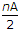# Electronics and Communication Engineering - Materials and Components

### Exercise :: Materials and Components - Section 3

46.

Assertion (A): An electron having charge - e and mass m and lying in an electric field E is subjected to an acceleration - (e/m) E.

Reason (R): If there are n electrons/m3 moving with velocity vx and each electron has a charge - e, the current density J = - n evx.

 A. Both A and R are true and R is correct explanation of A B. Both A and R are true but R is not correct explanation of A C. A is true but R is false D. A is false but R is true

Explanation:

No answer description available for this question. Let us discuss.

47.

When a pentavalent impurity is added, the semiconductor becomes

 A. p type B. n type C. intrinsic D. neutral

Explanation:

No answer description available for this question. Let us discuss.

48.

In body centred cubic structure, comers and centre of the cube are occupied by identical atoms.

 A. True B. False

Explanation:

No answer description available for this question. Let us discuss.

49.

For a multiplate capacitor having n plates, A as area of each plate, the capacitance is proportional to

 A. n A B. (n - 1) A C. (n + 1) A D.Explanation:

If the number of plates is n, the number of capacitors is (n - 1).

50.

The orientation polarization is

1. directly proportional to temperature
2. inversely proportional to temperature
3. inversely proportional to square of permanent dipole moment
4. directly proportional to square of permanent dipole moment.
Which of the above are correct?

 A. 1 and 3 only B. 2 and 3 only C. 1 and 4 only D. 2 and 4 only